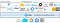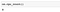# Why should you parallelize and distribute with Ray?While this tutorial explores how Ray makes it easy to parallelize plain Python code, it is important to note that Ray and its ecosystem also make it easy to parallelize existing libraries like scikit-learn, XGBoost, LightGBM, PyTorch, and much more. Image by Michael Galarnyk.

# How to get started with Ray

## Turning Python Functions into Remote Functions (Ray Tasks)

`pip install 'ray[default]'`
`import osimport timeimport ray# Normal Pythondef fibonacci_local(sequence_size):    fibonacci = []    for i in range(0, sequence_size):        if i < 2:            fibonacci.append(i)            continue        fibonacci.append(fibonacci[i-1]+fibonacci[i-2])    return sequence_size# Ray task@ray.remotedef fibonacci_distributed(sequence_size):    fibonacci = []    for i in range(0, sequence_size):        if i < 2:            fibonacci.append(i)            continue        fibonacci.append(fibonacci[i-1]+fibonacci[i-2])    return sequence_size`

## Comparing Local vs Remote Performance

`os.cpu_count()`The machine used in this tutorial has eight CPUs which means that each function below will generate 8 Fibonacci sequences.
`# Normal Pythondef run_local(sequence_size):    start_time = time.time()    results = [fibonacci_local(sequence_size) for _ in range(os.cpu_count())]    duration = time.time() - start_time    print('Sequence size: {}, Local execution time: {}'.format(sequence_size, duration))# Raydef run_remote(sequence_size):    # Starting Ray    ray.init()    start_time = time.time()    results = ray.get([fibonacci_distributed.remote(sequence_size) for _ in range(os.cpu_count())])    duration = time.time() - start_time    print('Sequence size: {}, Remote execution time: {}'.format(sequence_size, duration))`
`run_local(100000)run_remote(100000)`

## The Ray API

`fibonacci_distributed.remote(100000)`
`# To explicitly stop or restart Ray, use the shutdown APIray.shutdown()`

# Remote Objects as Actors

`from collections import namedtupleimport csvimport tarfileimport timeimport ray@ray.remoteclass GSODActor():    def __init__(self, year, high_temp):        self.high_temp = float(high_temp)        self.high_temp_count = None        self.rows = []        self.stations = None        self.year = year    def get_row_count(self):        return len(self.rows)    def get_high_temp_count(self):        if self.high_temp_count is None:            filtered = [l for l in self.rows if float(l.TEMP) >= self.high_temp]            self.high_temp_count = len(filtered)        return self.high_temp_count    def get_station_count(self):        return len(self.stations)    def get_stations(self):        return self.stations    def get_high_temp_count(self, stations):        filtered_rows = [l for l in self.rows if float(l.TEMP) >= self.high_temp and l.STATION in stations]        return len(filtered_rows)    def load_data(self):        file_name = self.year + '.tar.gz'        row = namedtuple('Row', ('STATION', 'DATE', 'LATITUDE', 'LONGITUDE', 'ELEVATION', 'NAME', 'TEMP', 'TEMP_ATTRIBUTES', 'DEWP',                                 'DEWP_ATTRIBUTES', 'SLP', 'SLP_ATTRIBUTES', 'STP', 'STP_ATTRIBUTES', 'VISIB', 'VISIB_ATTRIBUTES',                                 'WDSP', 'WDSP_ATTRIBUTES', 'MXSPD',                                  'GUST', 'MAX', 'MAX_ATTRIBUTES', 'MIN', 'MIN_ATTRIBUTES', 'PRCP',                                 'PRCP_ATTRIBUTES', 'SNDP', 'FRSHTT'))        tar = tarfile.open(file_name, 'r:gz')        for member in tar.getmembers():            member_handle = tar.extractfile(member)            byte_data = member_handle.read()            decoded_string = byte_data.decode()            lines = decoded_string.splitlines()            reader = csv.reader(lines, delimiter=',')            # Get all the rows in the member. Skip the header.            _ = next(reader)            file_rows = [row(*l) for l in reader]            self.rows += file_rows        self.stations = {l.STATION for l in self.rows}`
`# Code assumes you have the 1980.tar.gz and 2020.tar.gz files in your current working directory.def compare_years(year1, year2, high_temp):    """if you know that you need fewer than the default number of workers, you can modify the num_cpus parameter"""    ray.init(num_cpus=2)    # Create actor processes    gsod_y1 = GSODActor.remote(year1, high_temp)    gsod_y2 = GSODActor.remote(year2, high_temp)    ray.get([gsod_y1.load_data.remote(), gsod_y2.load_data.remote()])    y1_stations, y2_stations = ray.get([gsod_y1.get_stations.remote(),               	                    gsod_y2.get_stations.remote()])    intersection = set.intersection(y1_stations, y2_stations)    y1_count, y2_count = ray.get([gsod_y1.get_high_temp_count.remote(intersection),                                  gsod_y2.get_high_temp_count.remote(intersection)])    print('Number of stations in common: {}'.format(len(intersection)))    print('{} - High temp count for common stations: {}'.format(year1, y1_count))    print('{} - High temp count for common stations: {}'.format(year2, y2_count))#Running the code below will output which year had more extreme temperaturescompare_years('1980', '2020', 100)`

# Conclusion

::...#### 自怼圈/年度番新